# [Scala MOOC I] Lec2: Higher Order Functions

This week, we'll learn about functions as first-class values, and higher order functions. We'll also learn about Scala's syntax and how it's formally defined. Finally, we'll learn about methods, classes, and data abstraction through the design of a data structure for rational numbers.

## 2.1 - Higher-Order Functions

higher order functions: functions that takes functions as parameter or returns functions.

example: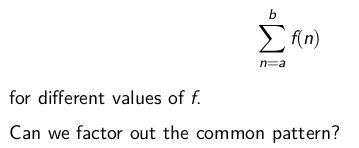⇒ put the `f` as a parameter

```def sum(f:Int => Int, a: Int, b: Int):Int = {
if(a>b) 0
else f(a) + sum(f, a+1, b)
}
```

### function types

`A => B` is a function that takes `A` as parameter and returns `B`.

### Anonymous functions

"literals" for functions, syntactic sugar. ex.

`(x: Int, y: Int) => x+y` `(x: Int) => x*x`

use anon functions in previous `sum()` function:

```sum(x=>x, 1, 10)
sum(x=>x*x, 1, 10)
```

exercice: turn `sum()` into tailrec fashion.

```def sum2(f:Int => Int, a: Int, b: Int):Int = {
@tailrec
def sumTR(a: Int, acc: Int): Int = {
if (a > b) acc
else sumTR(a + 1, acc + f(a))
}
sumTR(a, 0)
}
```

(note: using namescoping to eliminate parameters in inner functions)

## 2.2 - Currying

define a function that returns a funtion

```def sum3(f:Int => Int): (Int, Int)=>Int = {
def sumF(a:Int, b:Int):Int = {
if(a>b) 0
else f(a) + sum3(f, a+1, b)
}
sumF
}
```

when calling this function:
`sum3(cube)(1,10)`

syntactic sugar: shorter version of functions that return functions:

```def sum3(f:Int => Int)(a:Int, b:Int):Int = {
if(a>b) 0
else f(a) + sum3(f)(a+1, b)
}
```

question: what is type of `sum3`? → `Int => Int => (Int, Int) => Int` note: functional types are associated to the right,
`Int => Int => Int` is equivalent to `Int => (Int => Int)`

### "currying"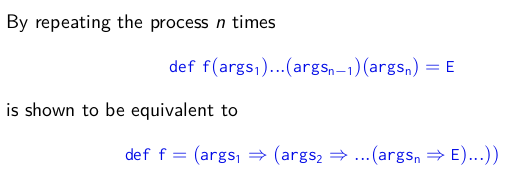exercice: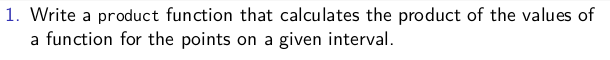```def product(f: Int => Int)(a: Int, b: Int): Int = {
if (a > b) 1
else a * product(f)(a + 1, b)
}
product(x=>x)(1,10)
```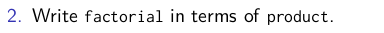```def fact(n:Int) = product(x=>x)(1,n)
fact(10)
```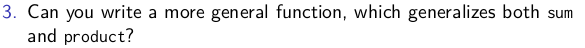def more_general(op: (Int,Int) => Int, default: Int) (f: Int=> Int)(a:Int, b:Int):Int = { if(a>b) default else op(a, more_general(op, default)(f)(a + 1, b)) } more_general((x,y)=>x+y, 0)(x=>x)(1,10)

def map_reduce(f:Int=> Int, op:(Int, Int)=>Int, default:Int) (a:Int, b:Int):Int = { if(a>b) default else op(f(a), map_reduce(f, op, default)(a+1,b)) } def factorial2(n:Int):Int = map_reduce(x=>x, (a,b)=> a*b, 1)(1,n) factorial2(10)

## 2.3 - Example: Finding Fixed Points

find the fix point of a function: x = f(x)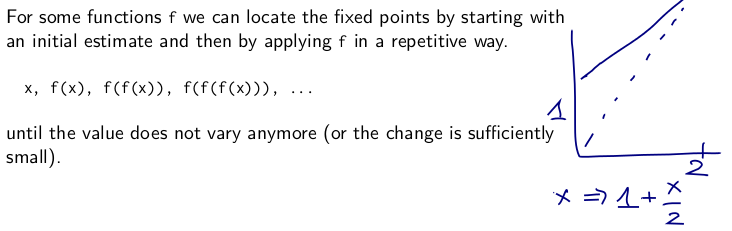```val tol = 0.001
def isCloseEnough(x:Double, y:Double):Boolean =
math.abs((x-y)/x)<tol
def fixedPoint(f: Double=>Double)(firstGuess:Double):Double = {
def iterate(guess:Double):Double = {
if(isCloseEnough(guess, f(guess))) guess
else iterate(f(guess))
}
iterate(firstGuess)
}
fixedPoint(x=>1+x/2)(1)
```

using the fixepoint function for `sqrt`:

• sqrt(x) = y such that: x=y*y
• =y such that y = x/y
• =fixed point for the function f(y)=x/y

def sqrt(x:Double):Double = fixedPoint(y=>x/y)(1) sqrt(2)

⇒ doesn't converge! ⇒ guess oscillates between 1 and 2... average damping: prevent the estimate from varying to much. ⇒ by taking the average of successive values

`def sqrt2(x: Double): Double = fixedPoint(y => (y + x / y) / 2)(1)`

abstract this damping technique:

```def avgDamping(f:Double=> Double)(x:Double):Double =
(f(x)+x)/2
def sqrt2(x: Double): Double = fixedPoint(avgDamping(y=>x/y))(1)
```

summary: The highest level of abstraction is not always the best, but it is important to know the techniques of abstraction, so as to use them when appropriate.

## 2.4 - Scala Syntax Summary

notations(EBNF):

• `|` denotes an alternative
• `[...]` an option (0 or 1)
• `{...}` a repetition (0 or more)

Types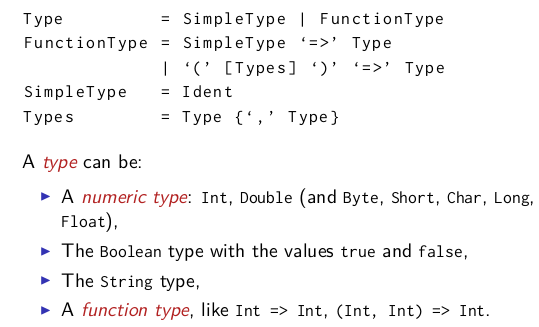Expressions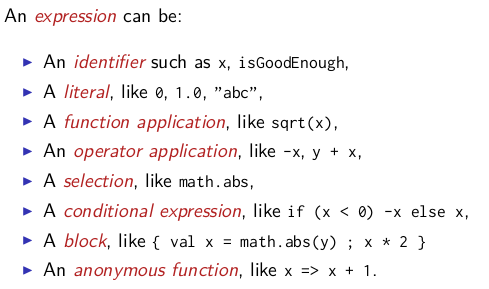Definitions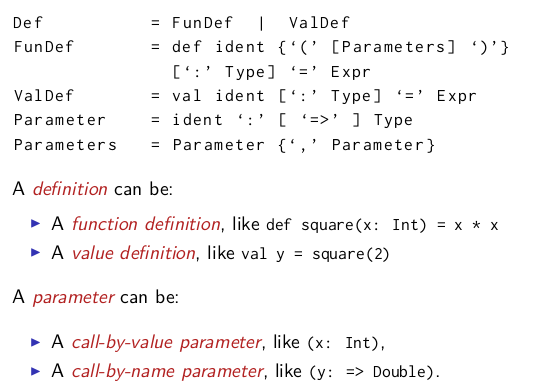## 2.5 - Functions and Data

example. rational numbers (x/y) define a class:

```class Rational(x:Int, y:Int){
def numer = x
def denom = y
}
val x = new Rational(1,2)
x.numer
x.denom
```

this definition creates both a class and the constructor.
now implement arithmetic:

```def add(that: Rational) =
new Rational(numer * that.denom + denom * that.numer, that.denom * denom)

def neg = new Rational(-numer, denom)

def sub(that: Rational) =

override def toString = numer + "/" + denom
```

## 2.6 - More Fun With Rationals

simplify the rationals at construction: add private members:

```private def gcd(a: Int, b: Int): Int = if (b == 0) a else gcd(b, a % b)
private val g = gcd(x, y)
def numer = x/g
def denom = y/g
```

other options:

• replace `g` with `gcd(x,y)`
• turn `numer` and `denom` into `val`

```def less(that:Rational) =
this.numer*that.denom < this.denom*that.numer

def max(that:Rational) = if(this.less(that)) that else this
```

### preconditions

ex: avoid divide by 0.

`require(y!=0, "denominator must be non zero")`

java.lang.IllegalArgumentException: requirement failed: denominator must be non zero

`require`is a test to perform when the class is initialized.
similar: `assert()`

### constructor

in scala a class implicitly introduces a primary constructor:

• takes parameters of the class
• executes all statements in the class body

`def this(x:Int) = this(x,1)`

exercice: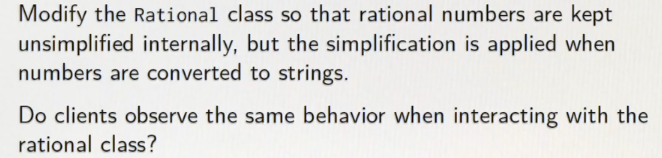```override def toString = {
val g = gcd(numer, denom)
numer/g + "/" + denom/g
}
```

## evaluation for class/object

extend the substitution model to classes and objects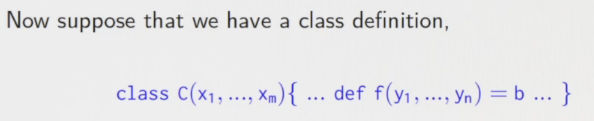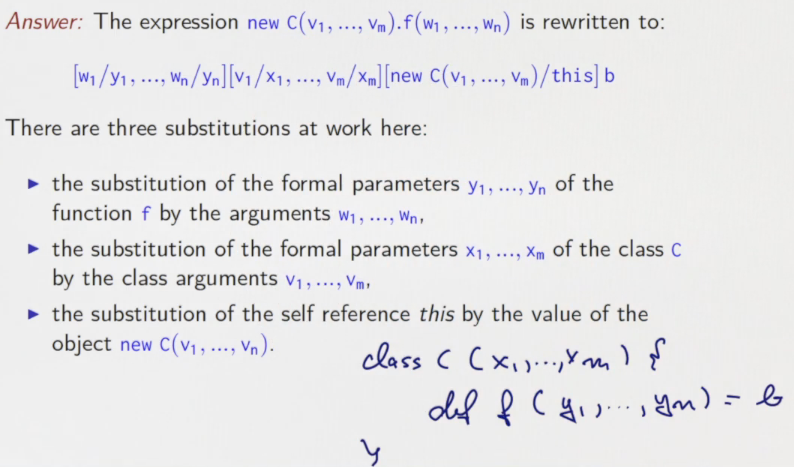examples:### infix ops

any method with one parameter can be used as an infix operator.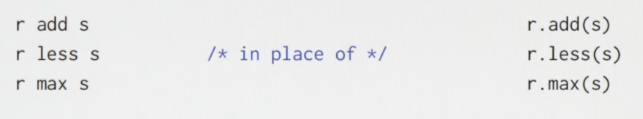scala identifiers can bu symbolic:⇒ change names to `+, <, -,` use in this way:

```x + y
x < y
x max y
x - y - z
```

### unitary ops

now change the `neg` method: prefix operator, and might be confused with the sub(`-`) ⇒ it's name is special: `unary_-`

`def unary_- = new Rational(-numer, denom)`

### precedence of ops

the precedence of an op is defined by its first letter order (by increasing precedence):quite the same as in java

## Programming Assignment: Functional Sets

Mathematically, we call the function which takes an integer as argument and which returns a boolean indicating whether the given integer belongs to a set, the characteristic function of the set. For example, we can characterize the set of negative integers by the characteristic function (x: Int) => x < 0.

Therefore, we choose to represent a set by its characterisitc function and define a type alias for this representation:

```type Set = Int => Boolean
def contains(s: Set, elem: Int): Boolean = s(elem)
```